# Constructing a Triangle with Sides of Three Given Lengths

Compass and straight edge constructions are of interest to mathematicians, not only in the field of geometry, but also in algebra. For thousands of years, beginning with the Ancient Egyptians, Indians and Greeks, mathematicians were interested in the problem of "duplicating the cube" (constructing a cube with twice the area of a given cube) using a straight edge and compass. It turns out that this is impossible, but no-one managed to prove this until 1837

However, it is possible to construct a triangle with sides of three different lengths using only a pair of compasses and a straight edge.

For this construction, you will need a straight edge (ruler - but you won't be measuring anything), pair of compasses, a pencil and paper. I have drawn the pictures using the robocompass app. It's fun to play with, and you can use it to do all sorts of geometric constructions. There's a little bit of coding to learn, but a list of instructions is provided. Once you've written your little program, you can get out the popcorn, sit back and watch the construction. Be warned that, when you are using it to draw arcs, the robocompass compass point may appear to be a little away from the centre, but the drawing is actually accurate.

## The Construction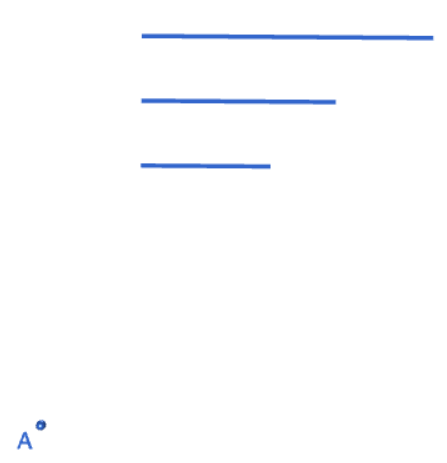Step 1: Start with three line segments. The lengths of these sides should be equal to the lengths of the sides of the triangle you wish to construct. Also draw a point $A$ that you want to use as one of the corners of your new triangle. Please don't be like Sam! Use a ruler to draw the line segments.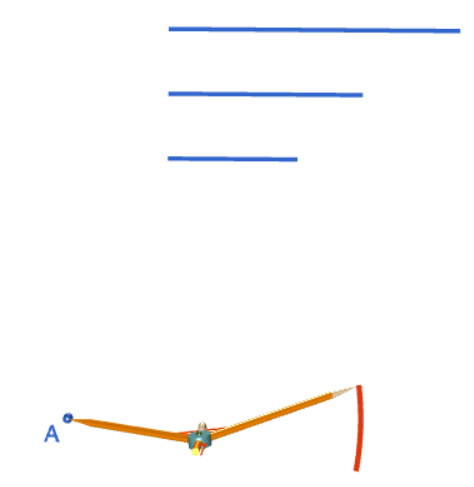Step 2: Open out your compasses to a radius that matches one of the line segments. Keeping this radius fixed, move your compasses down to point $A$ and make an arc near where you want the vertex $B$ of your triangle to lie. Draw a point $B$ somewhere on the arc. The line segment $AB$ will form the base of your triangle. The picture on the right shows what your construction should look like after you've completed this step.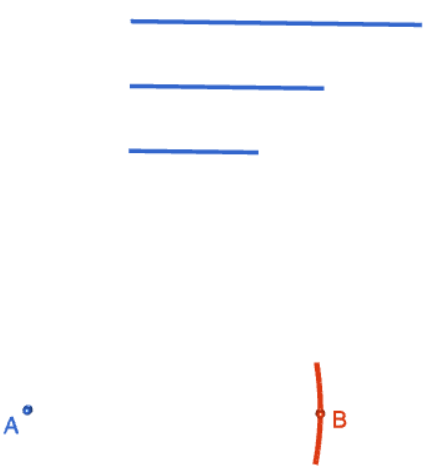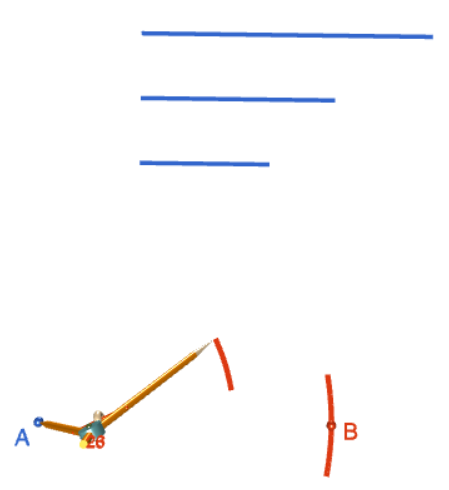Step 3: Open out your compasses to a radius that matches another one of the line segments. Keeping this radius fixed, move your compasses down to point $A$ and make an arc near where you want the vertex $C$ of your triangle to lie. All points of this arc are at the required distance away from $A$, but we don't know exactly where vertex $C$ will lie yet. The picture on the right shows the completed arc.Step 4: Open out your compasses to a radius that matches the final line segment. Move the tip of your compasses down to point $B$, and draw an arc that crosses the arc that you drew in step 3. The point where these two arcs cross will be vertex $C$ of your triangle. The picture on the right shows the completed arc.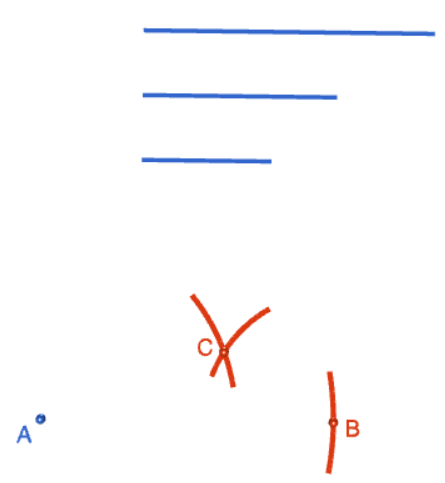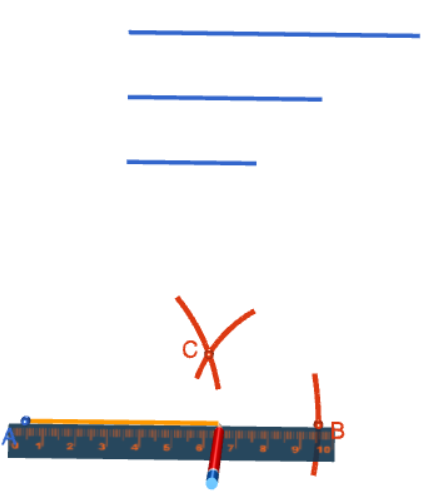Step 5: Finally, use your straight edge to join up the three vertices and make the triangle. Each side of the orange triangle has the same length as one of the three line segments that you started out with. The picture on the right shows the completed construction.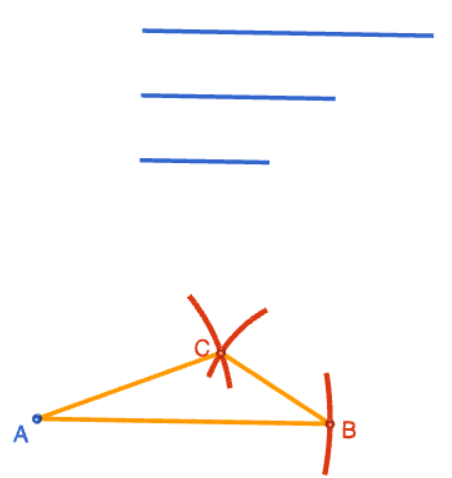### Description

This tutorial will expose you to Rulers and how to use them. We will also have a close look as set square and compass constructions. Your feedback is important to us, if you like any other topic covered under this tutorial, please do let us know.

### Audience

Year 10 or higher

### Learning Objectives

Ruler and compass constructions and more

Author: Subject Coach
You must be logged in as Student to ask a Question.